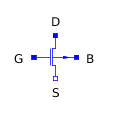Disk - MapleSim Help

Disk

Rigid diskDescription The Disk component models a homogeneous disk-like rigid body along a given axis with a predefined density. Based on the properties, i.e., axial unit vector, radius, and density, the center of mass, total mass, and moments of inertia are calculated for this rigid body. Although Disk and Cylinder components share similarities, Disk is preferred when length/thickness is insignificant compared to radius.Connections

 Name Description Modelica ID $\mathrm{frame__a}$ Center of disk frame_a $\mathrm{frame__c}\left[n\right]$ An array of additional frames on the circular cross section containing the center of mass frame_c[n]Parameters

 Name Default Units Description Modelica ID $\mathrm{e__axis}$ $\left[1,0,0\right]$ Axial unit vector e_axis $R$ $1$ $m$ Disk radius R $\mathrm{R__i}$ 0 m Disk inner radius R_i T 0.1 m Disk thickness T Select density Steel 7860 (kg/m^3) Select a predefined material density selectDensity $\mathrm{ρ}$ 1000 $\frac{\mathrm{kg}}{{m}^{3}}$ Disk user-defined material density customDensity Use additional frames false True means additional frames can be added addFrames $\mathrm{R__add}$ $\left[R\right]$ m Each value defines the radial distance of an additional frame to frame_a R_add[:] $\mathrm{θ__add}$  rad Each value defines the angle of rotation of an additional frame around the axial vector theta_add[:] Use initial conditions false True means parameters for specifying initial conditions for frame_a are enabled. Refer to: Rigid Body useICs Show visualization true True means the disk geometry is visible in the 3-D playback visualization Transparent false True means the geometry is transparent in the 3-D playback transparent ColorDisk color in the 3-D playback colorEquations The arrays and $\mathrm{θ__add}$ should have the same length. Each additional frame is defined by rotating frame_a around the axial vector an angle $\mathrm{θ__add}\left[i\right]$ and then translating along the reference axis by $\mathrm{L__add}\left[i\right]$. The reference axis is the next local axis after the e_axis (e.g., if e_axis is y, the reference axis is z). Figure 1 illustrates this process.Figure 1: The axial unit vector (e_axis) for this disk is [0,1,0]. Additional frame was added by defining and . Both of these frames lie on the plane defined by the normal vector of e_axis and passing through the center of mass.   Disk mass is calculated as where the disk material density, ρ, can be defined using the "Select density" parameter. This parameter lets the user either enter a value or select among predefined material densities.Figure 2: Different options for the "Select density" parameter   Assuming the default direction of $\left[1,0,0\right]$ for the e_axis, the moments of inertia expressed from the center of mass frame (frame_a) are $\mathrm{I__zz}=\mathrm{I__yy}$ The right-hand side of these equations will interchange if another axial unit vector is specified.ExamplesSpinning Top Figure 3 shows the layout of a model that uses a spherical joint, a Cylinder, and a Disk to simulate the precession of a spinning top. A snapshot of the 3-D playback is shown in Figure 4. This example also shows how the Cylinder and Disk components differ in modeling different rigid body geometries.Figure 3: Model layoutFigure 4: 3-D playback snapshotSlider-Crank Mechanism In this example, a Disk and three Cylinder components are connected with revolute and prismatic joints, as shown in Figure 5, to model a slider-crank mechanism. This model is similar to the one discussed in the Cylinder help page with the difference of using a Disk with an additional frame as the crank rather than a cylindrical rod. Using Disk and Cylinder components facilitates modeling by automatically calculating mass and moments of inertia and also results in realistic visualization in the 3-D playback window, as shown in Figure 6.Figure 5: Model layoutFigure 6: 3-D playback snapshot